Origami Cube
 What is the Origami Cube? Folding the Origami Cube Some Mathematics Origami Cube on the Internet References .
 To the Main Page        "Mathematische Basteleien"

What is the Origami Cube?
 ...The origami cube is a cube you can fold from a square sheet of paper. Another name is water bomb.

Folding the Origami Cube top
1Fold a square sheet of paper at the diagonals and unfold it. Put the paper at both arrows together. Lay the triangles at the top and at the bottom on top of each other.2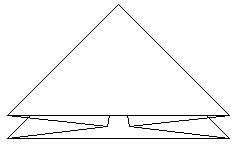The result is a flier triangle, which is known from the swallow or the pigeon.3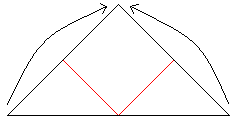Fold upward on the red line on the right and on the left.4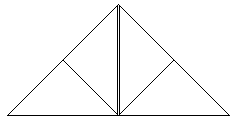Then it must look like this.5Fold on the red lines.6Then it must look like this.7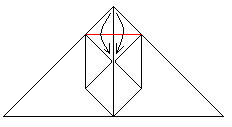Fold the two little triangles on the red lines downward.8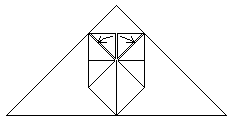Put the last made triangles in the two pockets on the right and on the left. This is a little bit fiddly.9Then it must look like this.10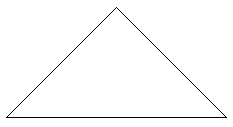Turn the whole triangle and repeat the steps 3,4,5,6,7,8,9.11Then it must look like this.12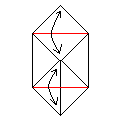Fold and unfold on the red lines.13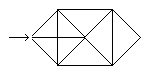Take the folded cube in hand and blow into the hole at the top. Unfold the cube by this.14finishedSome Mathematics top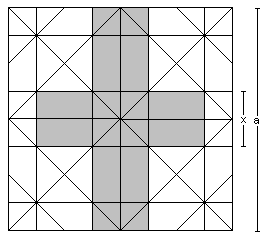If you take the cube apart, you get a nice pattern, which is made by the folds.   You find the formulas: .........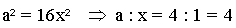On the left you can see the largest net of a cube, which fits a square. You find the formulas: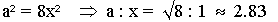This cube is much bigger than the folded cube.

If you like to use the whole square for a cube, you have the formula: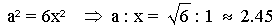This is the largest cube, theoretically.

See more in book (5), page 63f. and page 71ff.  "Der eingewickelte Würfel".

Origami Cube on the Internet

Englisch

Dr Stephen O'Hanlon (Ring of Origami Art)
Water Bomb  (  .pdf file)

Gerwin Sturm (Origami for everyone)
Waterbomb

Puzzle Playground  (Puzzle_com)
Square to Cube

Wikipedia
Water balloon

Deutsch

Jennifer's Puzzle
Flechten eines Würfels aus 3 Streifen

Umwelthaus Pinneberg e.V.
Wasserbombe aus Papier

Wikipedia
Wasserbombe (Spielzeug)

zzzebra
Wasserbombe

References   top
(1) Joachim Schönherr, Wir falten und falzen, Leipzig 1990
(2) Therese Mielhaht: Origami Spaß mit Papierfalten, Köln 1990
(3) Kunihiko Kasahara: Origami - figürlich und geometrisch, München 2000
(4) Kunihiko Kasahara: Origami - ohne Grenzen, München 2001
(5) Martin Gardner: Mathematische Hexereien, Berlin/Frankfurt am Main/Wien 1988 [ISBN 3550065787]

Feedback: Email address on my main page